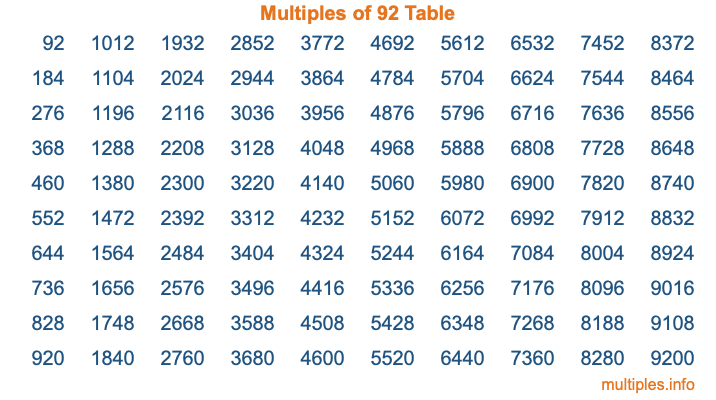Multiples of 92Welcome to the Multiples of 92 page. Here we will first teach you everything you will ever need to know about the multiples of 92, and then give you a study guide summary of everything we taught you to make sure you remember it all. Use this page to look up facts and learn information about the multiples of 92. This page will make you a multiples of ninety-two expert!

Definition of Multiples of 92
Multiples of 92 are all the numbers that when divided by 92 equal an integer. Each of the multiples of 92 are called a multiple. A multiple of 92 is created by multiplying 92 by an integer.

Therefore, to create a list of multiples of 92, you start with 1 multiplied by 92, then 2 multiplied by 92, then 3 multiplied by 92, and so on for as long as you want. Thus, the list of the first five multiples of 92 is 92, 184, 276, 368, and 460. To see a larger list of multiples of 92, see the printable image of Multiples of 92 further down on this page. We also have a category where you can choose any nth multiple of 92.

Multiples of 92 Checker
The Multiples of 92 Checker below checks to see if any number of your choice is a multiple of 92. In other words, it checks to see if there is any number (integer) that when multiplied by 92 will equal your number. To do that, we divide your number by 92. If the the quotient is an integer, then your number is a multiple of 92.

Is  a multiple of 92?

Least Common Multiple of 92 and ...
A Least Common Multiple (LCM) is the lowest multiple that two or more numbers have in common. This is also called the smallest common multiple or lowest common multiple and is useful to know when you are adding our subtracting fractions. Enter one or more numbers below (92 is already entered) to find the LCM.

Check out our LCM Calculator if you need more details about the Least Common Multiple or if you need the LCM for different numbers for adding and subtraction fractions.

nth Multiple of 92
As we stated above, 92 is the first multiple of 92, 184 is the second multiple of 92, 276 is the third multiple of 92, and so on. Enter a number below to find the nth multiple of 92.

th multiple of 92

Multiples of 92 vs Factors of 92
92 is a multiple of 92 and a factor of 92, but that is where the similarities end. All postive multiples of 92 are 92 or greater than 92. All positive factors of 92 are 92 or less than 92.

Below is the beginning list of multiples of 92 and the factors of 92 so you can compare:

Multiples of 92: 92, 184, 276, 368, 460, etc.

Factors of 92: 1, 2, 4, 23, 46, 92

As you can see, the multiples of 92 are all the numbers that you can divide by 92 to get a whole number. The factors of 92, on the other hand, are all the whole numbers that you can multiply by another whole number to get 92.

It's also interesting to note that if a number (x) is a factor of 92, then 92 will also be a multiple of that number (x).

Multiples of 92 vs Divisors of 92
The divisors of 92 are all the integers that 92 can be divided by evenly. Below is a list of the divisors of 92.

Divisors of 92: 1, 2, 4, 23, 46, 92

The interesting thing to note here is that if you take any multiple of 92 and divide it by a divisor of 92, you will see that the quotient is an integer.

Multiples of 92 Table
Below is an image of the first 100 multiples of 92 in a table. The table is in chronological order, column by column. The first column has the first ten multiples of 92, the second column has the next ten multiples of 92, and so on.The Multiples of 92 Table is also referred to as the 92 Times Table or Times Table of 92. You are welcome to print out our table for your studies.

Negative Multiples of 92
Although not often discussed or needed in math, it is worth mentioning that you can make a list of negative multiples of 92 by multiplying 92 by -1, then by -2, then by -3, and so on, to get the following list of negative multiples of 92:

-92, -184, -276, -368, -460, etc.

Multiples of 92 Summary
Below is a summary of important Multiples of 92 facts that we have discussed on this page. To retain the knowledge on this page, we recommend that you read through the summary and explain to yourself or a study partner why they hold true.

There are an infinite number of multiples of 92.

A multiple of 92 divided by 92 will equal a whole number.

92 divided by a factor of 92 equals a divisor of 92.

The nth multiple of 92 is n times 92.

The largest factor of 92 is equal to the first positive multiple of 92.

92 is a multiple of every factor of 92.

92 is a multiple of 92.

A multiple of 92 divided by a divisor of 92 equals an integer.

92 divided by a divisor of 92 equals a factor of 92.

Any integer times 92 will equal a multiple of 92.

Multiples of a Number
Here you can get the multiples of another number, all with the same attention to detail as we did for multiples of 92 on this page.

Multiples of
Multiples of 93
Did you find our page about multiples of ninety-two educational? Do you want more knowledge? Check out the multiples of the next number on our list!